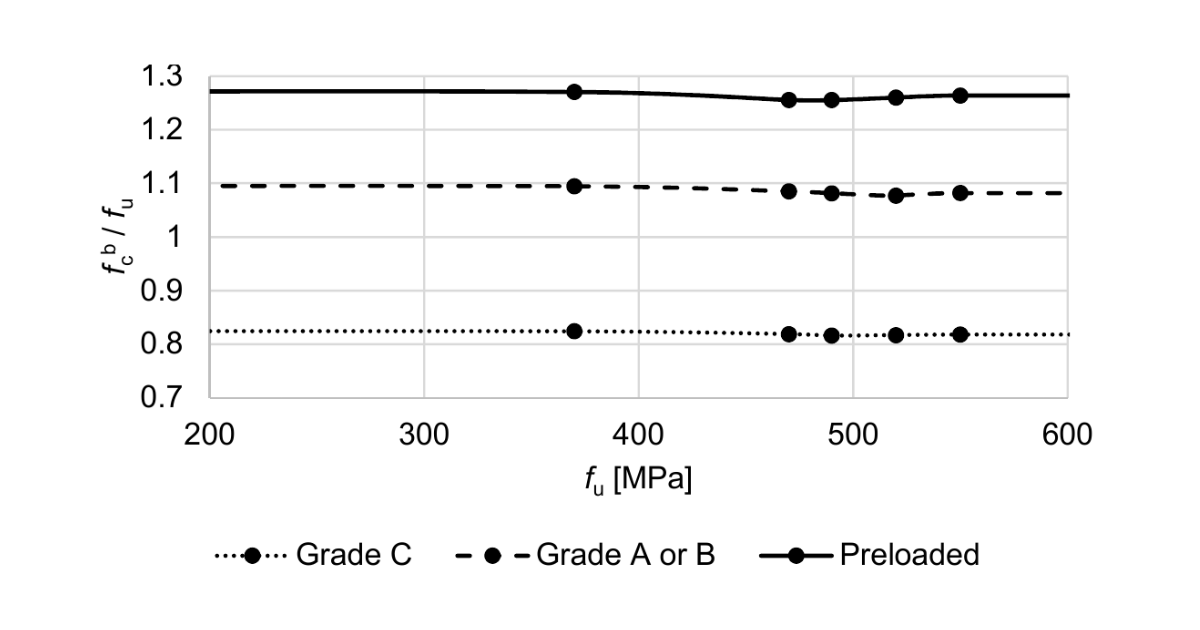### Choose language# Code-check of bolts according to Indian standard

Bolts are checked for shear, bearing, tension capacity and for combined tension and shear.
$$Shear capacity of boltsThe design strength of the bolt, $$V_{dsb}$$, as governed shear strength is given by IS 800, Cl. 10.3.3:$V_{sb} \le V_{dsb}$where: $$V_{dsb} = V_{nsb}/\gamma_{mb}$$ – design shear capacity of a bolt $$V_{nsb} = \frac{f_{ub}}{\sqrt{3}} A_e$$ – nominal shear capacity of a bolt $$f_{ub}$$ – ultimate tensile strength of a bolt; $$A_e$$ – area for resisting shear; $$A_e = A_n$$ for shear plane intercepted by the threads, $$A_e = A_s$$ for the case where threads do not occur in shear plane $$A_n$$ – net tensile stress area of the bolt $$A_s$$ – cross-section area at the shank $$\gamma_{mb} = 1.25$$ – partial safety factor for bolts – bearing type – IS 800, Table 5; editable in Code setup When the grip length of bolts $$l_g$$ (equal to the total thickness of the connected plates) is higher than $$5d$$, the design shear capacity $$V_{dsb}$$ is reduced by a factor $$\beta_{lg}$$ – IS 800, Cl. 10.3.3.2:$\beta_{lg} = \frac{8}{3+l_g/d}$According to IS 800, Cl. 10.3.3.3, the design shear capacity of bolts carrying shear through a packing plate with the thickness $$t_{pk} \ge 6$$ mm shall be decreased by a factor:$\beta_{pk} = (1-0.0125 t_{pk})$Each shear plane is checked separately, and the worst result is shown.Bearing capacity of boltsThe design bearing strength of a bolt on any plate, as governed by bearing is given by IS 800, Cl. 10.3.4:$V_{sb} \le V_{dpb}$where: $$V_{dpb} = V_{npb} / \gamma_{mb}$$ – design bearing strength of a bolt $$V_{npb} = 2.5 k_b d t f_u$$ – nominal bearing strength of a bolt $$k_b = \min \left \{ \frac{e}{3d_0}, \, \frac{p}{3d_0}-0.25, \, \frac{f_{ub}}{f_u}, \, 1.0 \right \}$$ – factor for joint geometry and material strength $$e$$ – end distance of the fastener along bearing direction $$p$$ – pitch distance of the fastener along bearing direction $$f_{ub}$$ – ultimate tensile strength of the bolt $$f_u$$ – ultimate tensile strength of the plate $$d$$ – nominal diameter of the bolt $$d_0$$ – diameter of bolt hole $$t$$ – plate thickness $$\gamma_{mb} = 1.25$$ – partial safety factor for bolts – bearing type – IS 800, Table 5; editable in Code setup Bearing on each plate is checked separately and the worst result is shown.The bearing resistance is reduced for oversized and slotted holes by a factor: 0.7 – for oversized and short slotted holes 0.5 – for long slotted holes Sizes of oversized, short slotted, and long slotted holes are determined according to IS 800, Table 19.Tension capacity of boltsA bolt subjected to a factored tensile force is checked according to IS 800, Cl. 10.3.5:$T_b \le T_{db}$where: $$T_{db} = T_{nb} / \gamma_{mb}$$ – design tensile capacity of the bolt $$T_{nb} = \min \{ 0.9 f_{ub} A_n, \, f_{yb} A_s (\gamma_{mb} / \gamma_{m0}) \}$$ – nominal tensile capacity of the bolt $$f_{ub}$$ – ultimate tensile strength of the bolt $$f_{yb}$$ – yield strength of the bolt $$A_n$$ – net tensile stress area of the bolt $$A_s$$ – cross-section area at the shank $$\gamma_{mb} = 1.25$$ – partial safety factor for bolts – bearing type – IS 800, Table 5; editable in Code setup $$\gamma_{m0} = 1.1$$ – partial safety factor for resistance governed by yielding – IS 800, Table 5; editable in Code setup Bolt subjected to combined shear and tensionA bolt required to resist both design shear force and design tensile force at the same time shall according to IS 800, Cl. 10.3.6 satisfy:$\left( \frac{V_{sb}}{V_{db}} \right)^2 + \left( \frac{T_{b}}{T_{db}} \right)^2 \le 1.0$where: $$V_{sb}$$ – factored shear force $$V_{db} = \min \{ V_{dsb}, \, V_{dpb} \}$$ – design shear resistance of the bolt – IS 800, Cl. 10.3.2 $$V_{dsb}$$ – design shear resistance $$V_{dpb}$$ – design bearing resistance $$T_b$$ – factored tensile force $$T_{db}$$ – design tensile capacity of the bolt$$